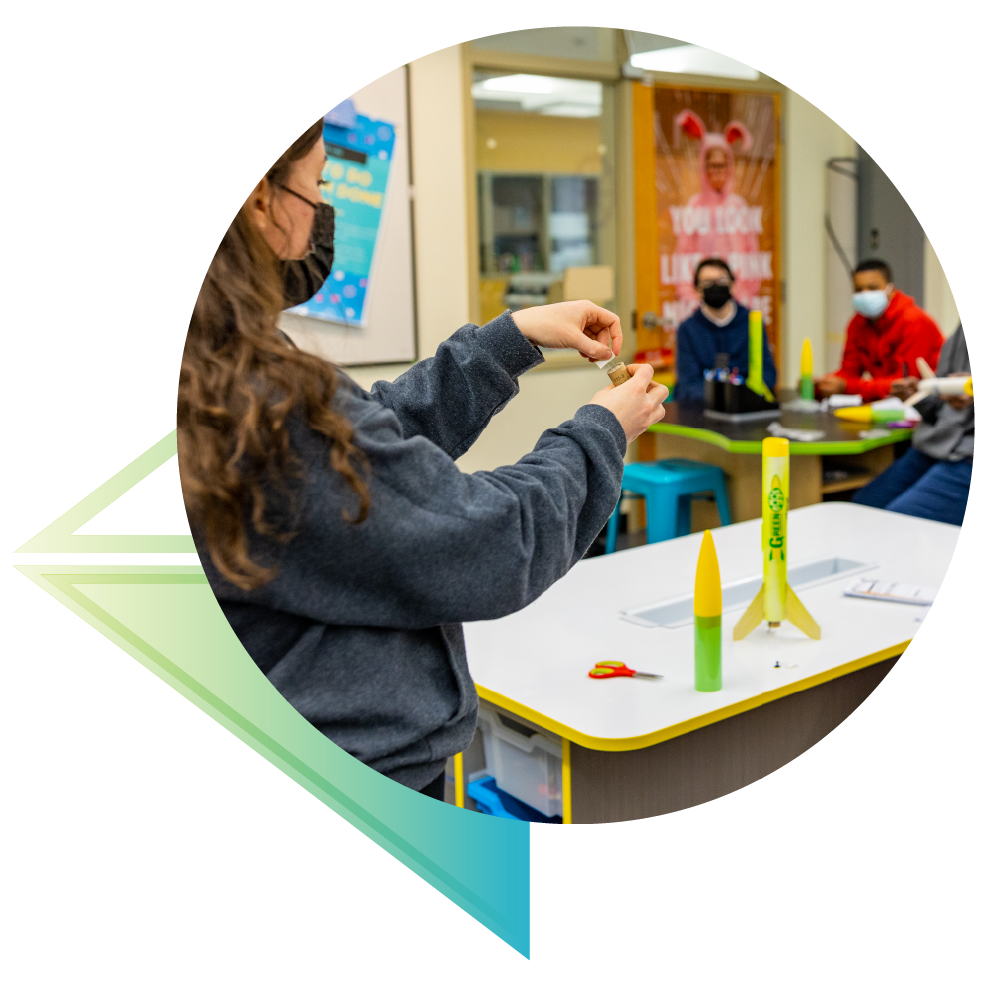# Slippery Slope - Unit Plan

## Slippery Slope - Unit Plan

View full details

## Grades 6-8 | 10 (45 min) Classes

In this unit students will answer the question: How is slope represented in a model rocket launch?

Students will learn about the different types of slope and practice calculation methods to find slope from a graph. Students will then analyze launch data to identify and calculate where a rocket has a positive, negative and/or neutral slope on its flight path.

For their final assessment students will be creating a visual of the four types of slope that shows how slope is seen on a model rocket’s flight path.

## Common Core Standards - Math

### CCSS.MATH.CONTENT.7.RP.A.2

Recognize and represent proportional relationships between quantities.

### CCSS.MATH.CONTENT.7.RP.A.2.A

Decide whether two quantities are in a proportional relationship.

### CCSS.MATH.CONTENT.7.RP.A.2.B

Identify the constant of proportionality (unit rate) in tables, graphs, equations, diagrams, and verbal descriptions of proportional relationships.

### CCSS.MATH.CONTENT.8.F.A.2

Compare properties of two functions each represented in a different way (algebraically, graphically, numerically in tables, or by verbal descriptions).

### CCSS.MATH.CONTENT.8.F.A.3

Interpret the equation y = mx + b as defining a linear function, whose graph is a straight line; give examples of functions that are not linear.

### CCSS.MATH.CONTENT.8.F.B.5

Describe qualitatively the functional relationship between two quantities by analyzing a graph (e.g., where the function is increasing or decreasing, linear or nonlinear). Sketch a graph that exhibits the qualitative features of a function that has been described verbally.

## High School

### CCSS.MATH.CONTENT.HSF.IF.B.4

For a function that models a relationship between two quantities, interpret key features of graphs and tables in terms of the quantities, and sketch graphs showing key features given a verbal description of the relationship

### CCSS.MATH.CONTENT.HSS.ID.C.7

Interpret the slope (rate of change) and the intercept (constant term) of a linear model in the context of the data.

## Building Supplies## Live, Online Training and 1:1 Support!

Estes Education has been a leader in STEM education for over 60 years. We know that STEM is a vital interdisciplinary topic that requires hands-on and inclusive learning. Explore these supporting materials to take learning to new heights!

Should you have need, you can meet with a member of Education team for FREE, 1:1 online support to learn rocketry basics, how to apply our curriculum, and discover unique teaching strategies. Our team is eager to answer your questions!## Learn About Model Rocket Safety!

Not sure how to safely launch a rocket with your group? Head over to our dedicated Safety instructions page for videos, support, and more!